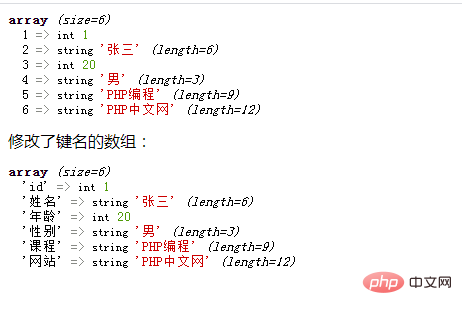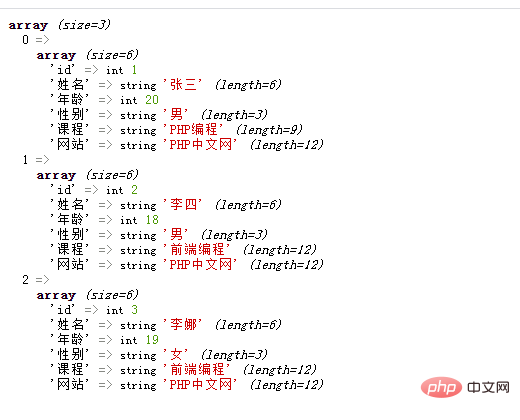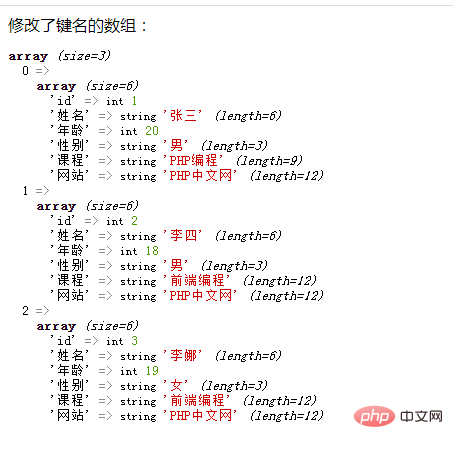# PHP数组学习之改变一维和二维数组的键key

→相关推荐：PHP数组学习系列大汇总（持续更新~）

```<?php
\$arr=array(1 => 1, 2 => "张三", 3 => 20, 4 => "男", 5 => 'PHP编程', 6 => 'PHP中文网');
\$key=array("id","姓名","年龄","性别","课程","网站");
\$array=array_combine(\$key, \$arr);
var_dump(\$arr);
echo "修改了键名的数组：";
var_dump(\$array);
?>```

array_combine() 函数通过合并两个数组来创建一个新数组，其中的一个数组元素为键名，另一个数组的元素为键值。注：两个数组中元素个数必须要一致，这才能让键名和键值一一对应，否则就会报错并返回 FALSE。【推荐文章《PHP数组学习之如何以合并方式创建数组》】```<?php
\$arr=array(
array(1 => 1, 2 =>"张三", 3 => 20, 4 => "男", 5 => 'PHP编程', 6 => 'PHP中文网'),
array(1 => 2, 2 =>"李四", 3 => 18, 4 => "男", 5 => '前端编程', 6 => 'PHP中文网'),
array(1 => 3, 2 =>"李娜", 3 => 19, 4 => "女", 5 => '前端编程', 6 => 'PHP中文网')
);
\$key=array("id","姓名","年龄","性别","课程","网站");
foreach(\$arr as \$k=>\$v){
\$arr[\$k] = array_combine(\$key, \$v);
}
var_dump(\$arr);
?>``````<?php
\$arr=array(
array(1 => 1, 2 =>"张三", 3 => 20, 4 => "男", 5 => 'PHP编程', 6 => 'PHP中文网'),
array(1 => 2, 2 =>"李四", 3 => 18, 4 => "男", 5 => '前端编程', 6 => 'PHP中文网'),
array(1 => 3, 2 =>"李娜", 3 => 19, 4 => "女", 5 => '前端编程', 6 => 'PHP中文网')
);

\$key=array("id","姓名","年龄","性别","课程","网站");

function foo(&\$v, \$k, \$key) {
\$v = array_combine(\$key, array_slice(\$v, 0));
}
array_walk(\$arr, 'foo', \$key);

echo "修改了键名的数组：";
var_dump(\$arr);
?>```

array_walk() 函数对数组中的每个元素应用用户自定义函数。在函数中，数组的键名和键值是参数。### 全部评论我要评论

• 取消发布评论发送
• 1/1